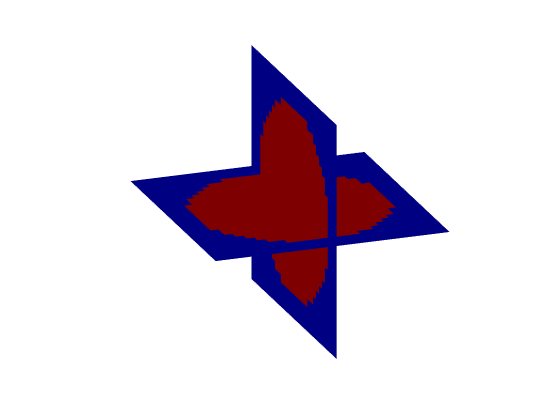beamPlot

Plot volumetric data using intersecting planes.

Syntax

beamPlot(mat)
beamPlot(mat, plot_yz)
beamPlot(xy_slice, xz_slice)

Description

beamPlot plots 3D volumetric data as intersecting planes using slice. The data is assumed to be indexed as (x, y, z). The central x-y and x-z planes are extracted and plotted as intersecting planes. The first y-z plane can also be plotted by setting the optional input plot_yz to true. This orientation is useful for plotting the beam patterns produced by ultrasound transducers facing in the x-direction.

beamPlot can alternatively be called with two 2D slices which are plotted as intersecting planes indexed as (x, y) and (x, z). The slices must have the same size in the x-direction.

Examples:

beamPlot(makeBall(30, 30, 30, 15, 15, 15, 12));
beamPlot(makeDisc(40, 30, 20, 15, 10), makeDisc(40, 20, 20, 10, 5));Inputs

 mat 3D matrix to plot plot_yz Boolean controlling whether the first y-z plane is displayed xy_slice slice to plot in the x-y plane xz_slice slice to plot in the x-z plane